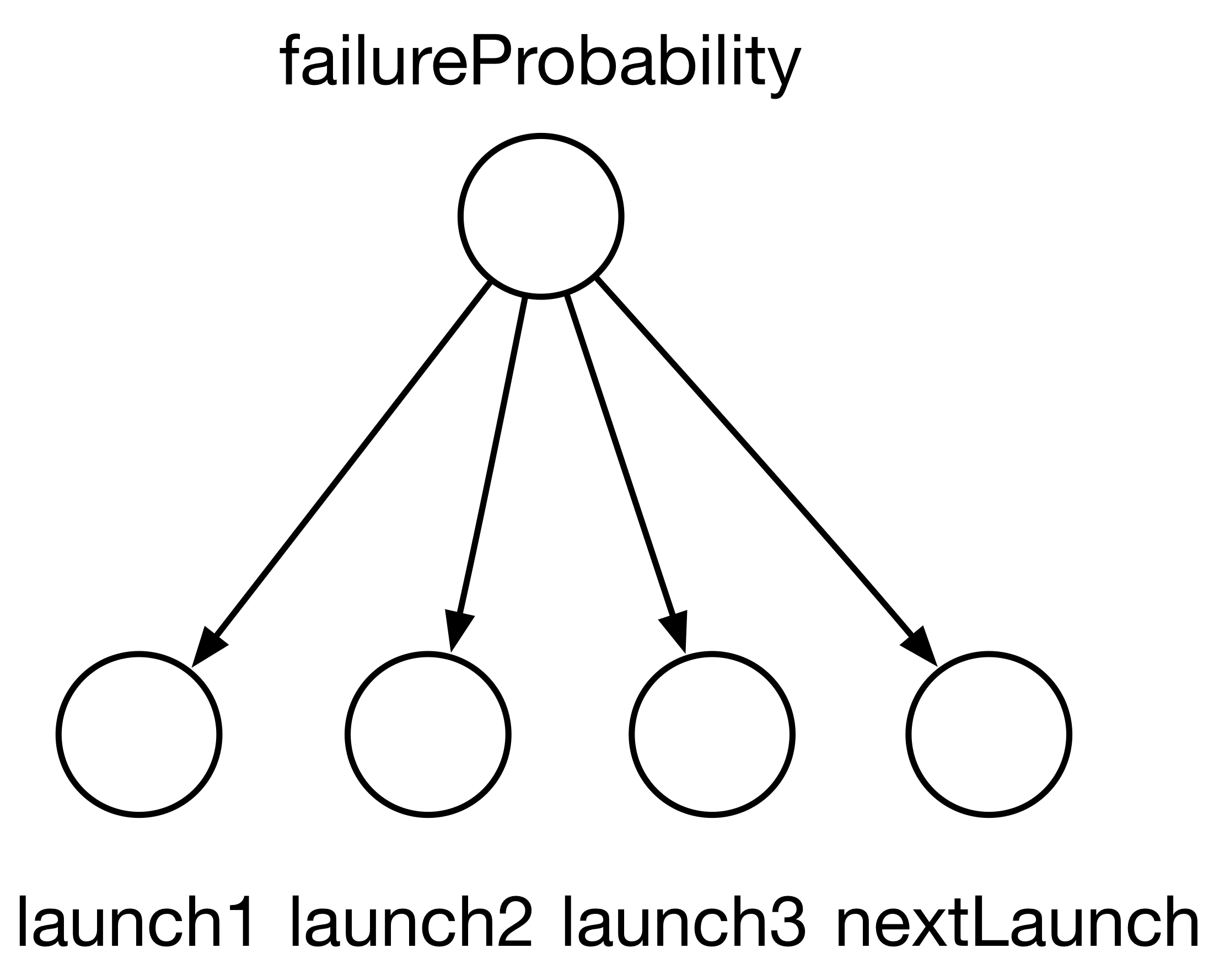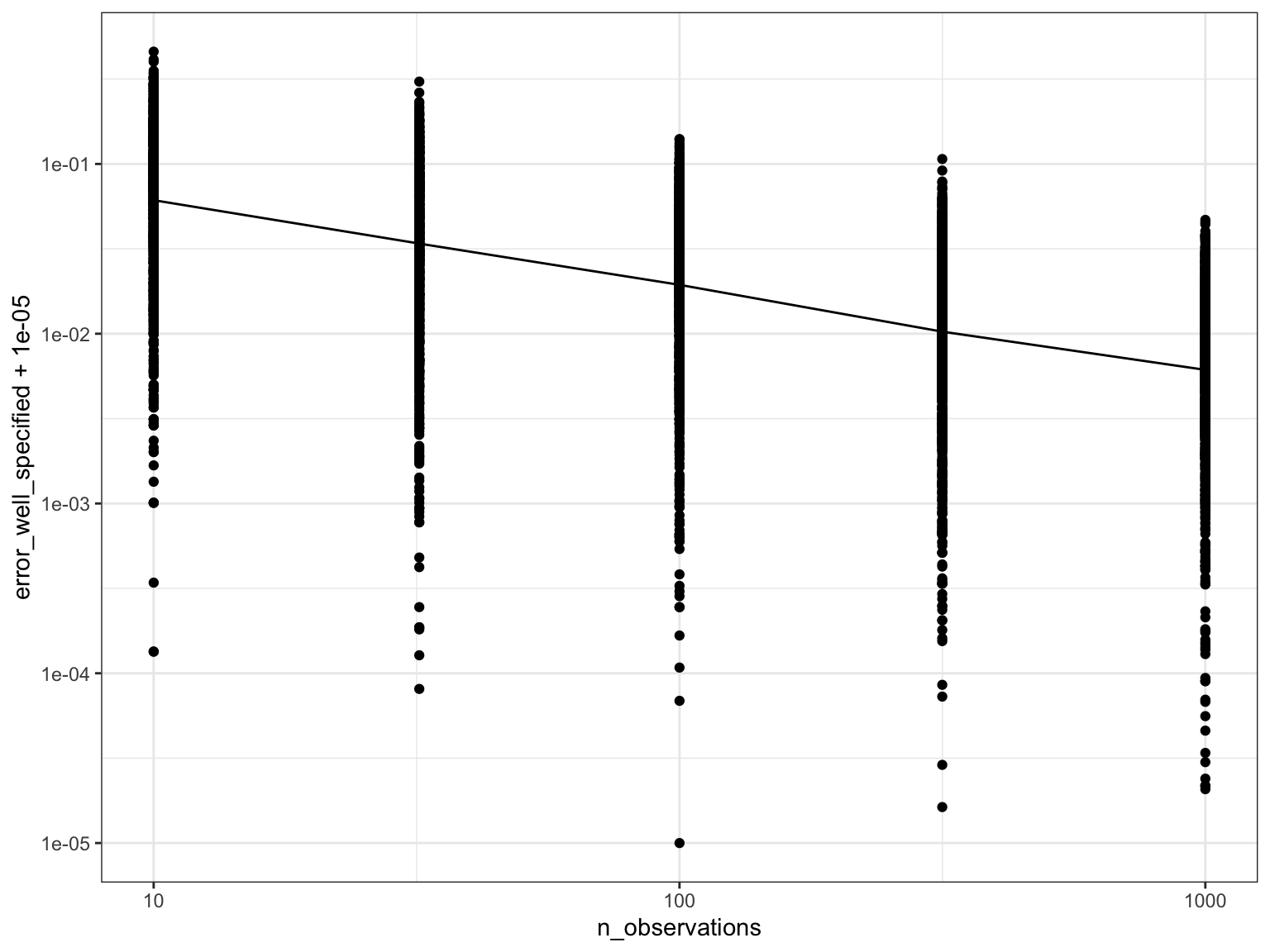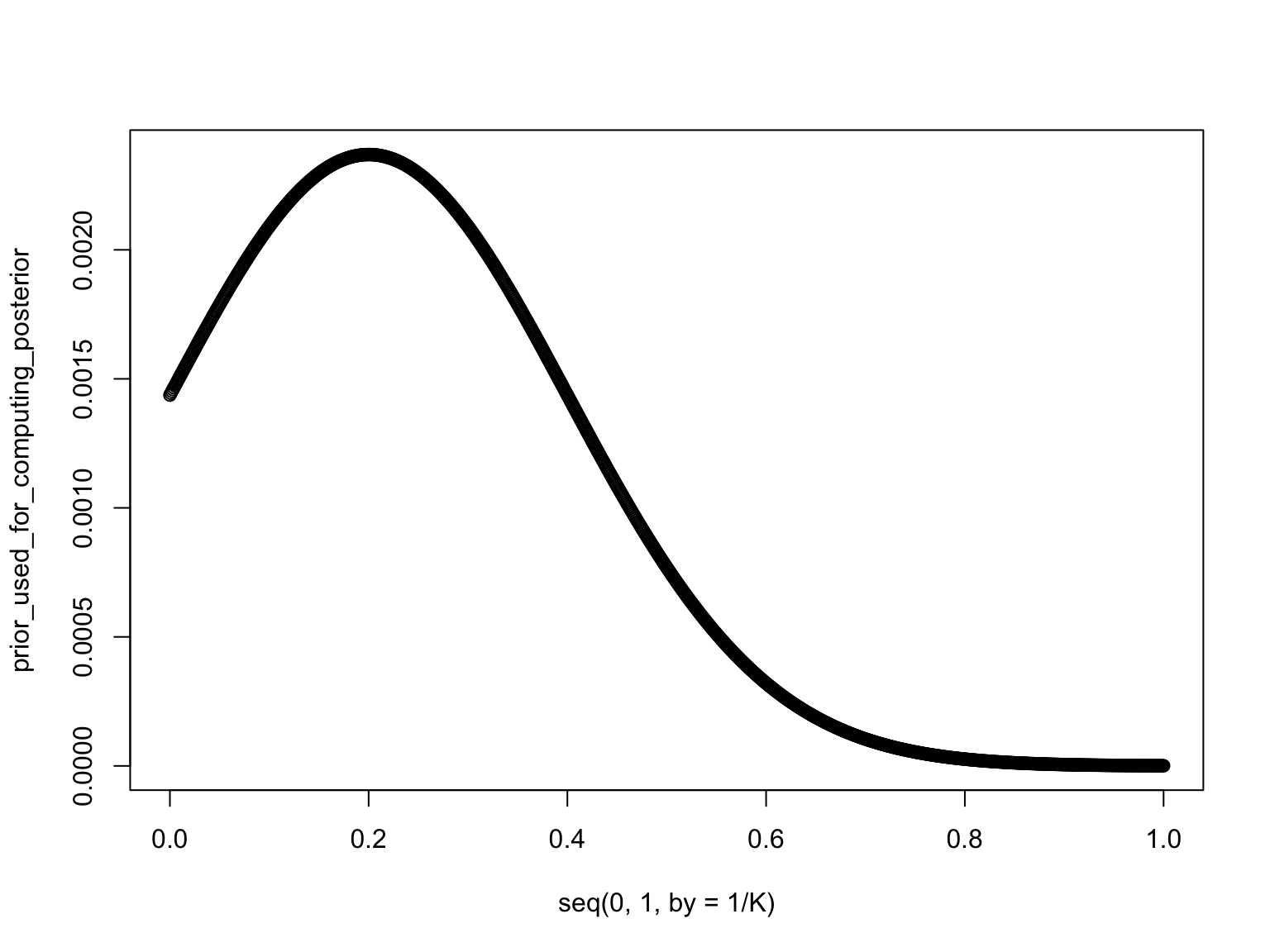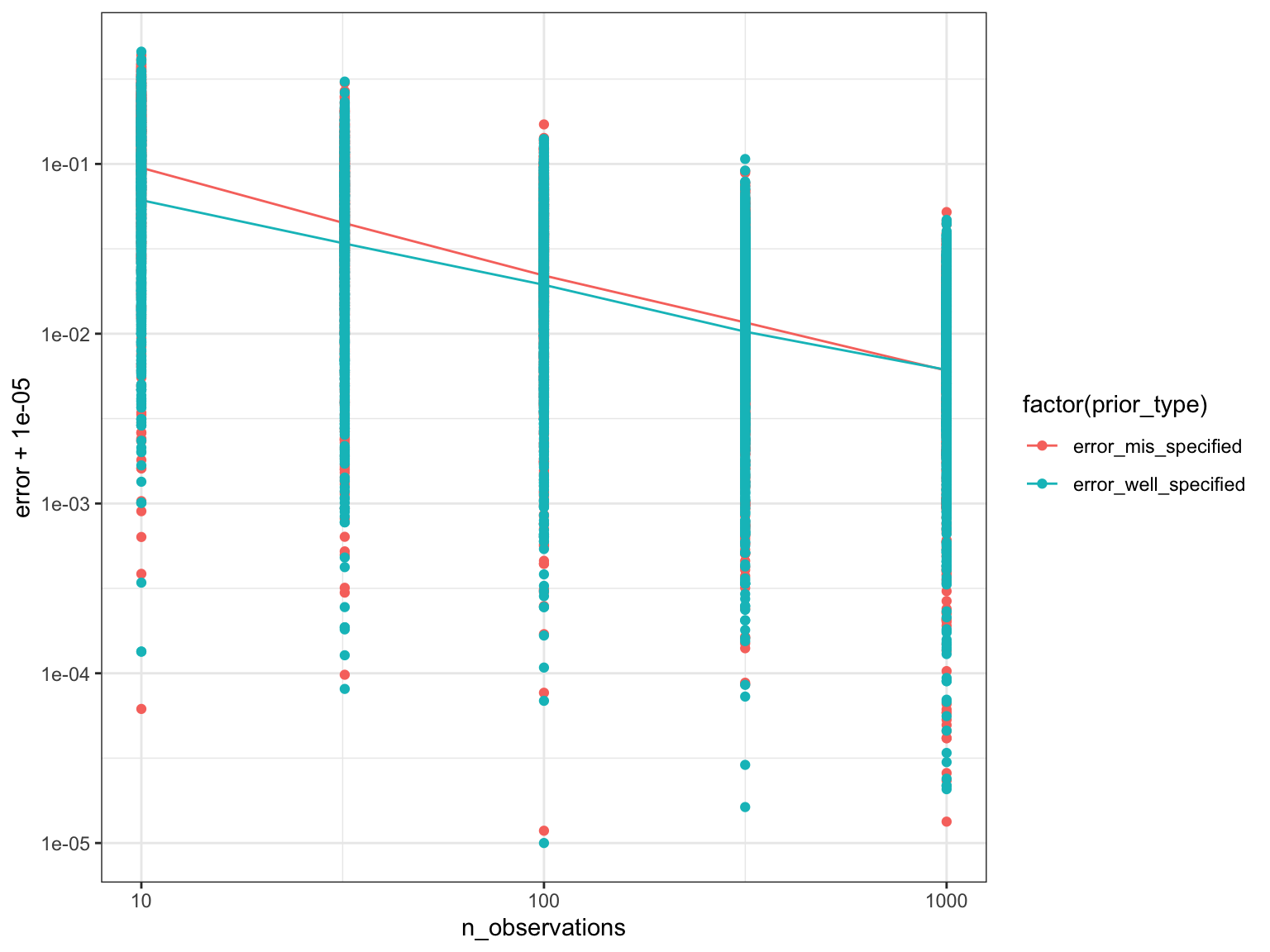# Accuracy of point estimates as a function of number of observations

• You are estimating some parameter of interest.
• You are asked for one more significant digit in your point estimate.

### Poll: how many additional observations should you collect?

1. 10 times more data
2. 100 times more data
3. 1000 times more data
4. there is no way to answer this question

# Back of the envelope calculation

• Frequentist world: if the estimator $$\delta = \frac{1}{n} \sum_{i=1}^n X_i$$ is an empirical average of iid random variables
• Getting one more significant digit consists in decreasing the standard deviation (SD) of $$\delta$$ by factor 10

$\text{SD}(\delta) = \sqrt{{\text{Var}}\frac{1}{n} \sum_{i=1}^n X_i} = \sqrt{\frac{n {\text{Var}}X_1}{n^2}} = \frac{\text{constant}}{\sqrt{n}}$

• Hence, to decrease SD by factor 10, we need to increase $$n$$ by factor 100
• Today: what if $$\delta$$ is a posterior mean?

# Example

• Setup: posterior mean of the parameter $$p$$ of a Bernoulli conditioning on $$n$$ observations
• Similar to the delta rocket/earth-water problems
• But let us simplify the problem further: use a discrete prior on $$p$$, with point masses at $$\{0, 1/K, 2/K, \dots, 1\}$$
• This way we can do simulations very quickly based on arbitrary priors
• But we will see how our findings on this simple model can be generalized to other exchangeable models# Numerical exploration

• Code to compute posterior distribution and posterior mean
posterior_distribution <- function(prior_probs, n_successes, n_trials){
K <- length(prior_probs)-1 # K+1 values that your p can assume
n_fails <- n_trials - n_successes
p <- seq(0, 1, 1/K)
posterior_probs <-                                       # 1. this computes gamma(i)
prior_probs *                                          #    - prior
p^n_successes * (1-p)^n_fails                          #    - likelihood
posterior_probs <- posterior_probs/sum(posterior_probs)  # 2. normalize gamma(i)
post_prob <- rbind(p, posterior_probs)
return(post_prob)
}

posterior_mean <- function(posterior_probs){
return(sum(posterior_probs[1,] * posterior_probs[2,]))
}
• Code to:
1. generate $$p$$
2. generate a dataset based on $$p$$
3. compute posterior mean based on data
4. measure error between generated $$p$$ and posterior mean
set.seed(1)
rdunif <- function(max) { return(ceiling(max*runif(1))) } # unif 1, 2, .., max
K = 1000

# Later, we will use different prior to generate data versus do inference,
# but for now, use the same (uniform on the K+1 grid points)
prior_used_for_computing_posterior <- rep(1/(K+1), K+1)

simulate_posterior_mean_error <- function(n_datapoints) {
i <- rdunif(K + 1) - 1  # Always generate the data using a uniform prior
true_p <- i/K
x <- rbinom(1, n_datapoints, true_p) # Recall: a Binomial is just a sum of iid Bernoullis
post <- posterior_distribution(prior_used_for_computing_posterior, x, n_datapoints)
post_mean <- posterior_mean(post)
return(abs(post_mean - true_p)) # Return the error by comparing posterior mean and p used to generate data
}

# Accuracy of point estimate: empirical results

• Calling our new function to get errors for:
• 1000 datasets each of size 10 (= 10^1)
• 1000 datasets each of size 32 (= 10^(3/2))
• 1000 datasets each of size 100 (= 10^2)
set.seed(1)
df <- data.frame("n_observations" = rep(c(10, 32, 100, 316, 1000), each=1000))
df$error_well_specified <- sapply(df$n_observations, simulate_posterior_mean_error)

ggplot(data=df, aes(x=n_observations, y=error_well_specified+1e-5)) +
stat_summary(fun.y = mean, geom="line") + # Line averages over 1000 datasets
scale_x_log10() +  # Show result in log-log scale
scale_y_log10() +
geom_point()• Good news: error goes to zero
• This property is called consistency
• Viewed as bare minimum of statistical procedures
• But here we are interested in the rate (how fast it goes to zero?)
• Result suggests a linear fit in the log-log scale $$\underbrace{\log_{10}(\text{error})}_{y} = a\; \underbrace{\log_{10}(\text{number of observation})}_{x} + b$$

• Eyeball the coefficient $$a = \Delta x / \Delta y$$

1. 2
2. 1/2
3. 1
4. -1/2
5. -2

# Accuracy of point estimate as a function of number of observations

• Get $$a \approx -1/2$$

• Hence taking power on each side of

$$\underbrace{\log_{10}(\text{error})}_{y} = a \underbrace{\log_{10}(\text{number of observation})}_{x} + b$$

we get $$\text{error} = O((\text{number of observations})^{-1/2})$$

• Similarly to the frequentist setting, for many models, root mean square error will scale like $\frac{\text{constant}}{\sqrt{\text{number of observations}}}$

• So if we want to reduce it by factor 10 (i.e. “gain one significant digit”), need 100x more observations
• To gain 2 more significant digits: need 10,000x more observations, etc

• What we did here is an example of an (empirical) asymptotic analysis

# What if we used the “wrong” prior

• Recall the non-uniform prior from the exercise question:
prior_used_for_computing_posterior <- dnorm(seq(0, 1, 1/K),mean = 0.2, sd=0.2)
prior_used_for_computing_posterior <- prior_used_for_computing_posterior / sum(prior_used_for_computing_posterior)
plot(seq(0, 1, by=1/K), prior_used_for_computing_posterior)• The code below will add another curve to the error plot
• In this new curve, the posterior is computed based on the wrong (non-uniform) prior
• But we still simulate the “true” $$p$$ uniformly, hence the misspecification
set.seed(2)
df$error_mis_specified <- sapply(df$n_observations, simulate_posterior_mean_error)
df <- gather(data=df, key=prior_type, value=error, error_mis_specified, error_well_specified)

ggplot(data=df, aes(x=n_observations, y=error+1e-5, colour=factor(prior_type))) +
stat_summary(fun.y = mean, geom="line") +
scale_x_log10() +
scale_y_log10() +
geom_point()• The misspecified model exhibits slightly lower performance when there are 10 observations,
• but the performance of the two models become very close as the number of observations increases
• Do you think this will always be like this for any misspecified models?

# First way to break consistency: violating Cromwell’s rule

• Suppose we put zero prior mass to all $$p$$ smaller than $$1/2$$. What happens on the posterior?

• If we put prior mass of zero to some event, say $$\pi_0(A) = 0$$ then the posterior probability of that event will always be zero, $$\pi_n(A) = 0$$, no matter how many observations $$n$$ we get

• Cromwell’s rule (Oliver Cromwell, 1650): “I beseech you, in the bowels of Christ, think it possible that you may be mistaken.”

# Second way to break consistency: Unidentifiable models

• When there are too many parameters, you may not be able to recover them even if you had “infinite data”

• Example:
• Let us modify the prior for the rocket example (continuous version), setting $$p = p_1 p_2$$ where $$p_1 \sim {\text{Unif}}(0,1)$$ and $$p_2 \sim {\text{Unif}}(0,1)$$.
• Intuition: there are pairs of $$(p_1, p_2)$$ that yield the same joint density, e.g. $$(p_1, p_2) = (1, 0.2)$$ and $$(p_1, p_2) = (0.5, 0.4)$$.
• Model code in the Blang PPL

model Unidentifiable {
...
laws {
p1 ~ ContinuousUniform(0, 1)
p2 ~ ContinuousUniform(0, 1)
nFails | p1, p2, nLaunches ~ Binomial(nLaunches, p1 * p2)
}
}
• Results